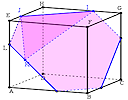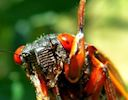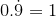Search IntMath
Close

450+ Math Lessons written by Math Professors and Teachers

5 Million+ Students Helped Each Year

1200+ Articles Written by Math Educators and Enthusiasts

Simplifying and Teaching Math for Over 23 Years

#### Tips, tricks, lessons, and tutoring to help reduce test anxiety and move to the top of the class.

By Murray Bourne, 30 Apr 2013

30 Apr 2013

1. Math resource: MathGraph32
3. Relieving test anxiety
4. Math puzzle
5. IntMath Poll
6. Final thought:

Once again I apologize for the long gap since the last IntMath Newsletter. I'm involved in several large projects (including developing online modules for a math course and 2 academic integrity courses). Once those are done, hopefully I'll be able to get back to a regular schedule of writing.

## 1. Math resource: MathGraph32MathGraph32 is a great free tool for exploring 2D and 3D math concepts. MathGraph32The 17-year cicada is due to emerge in north-eastern parts of USA in Spring 2013. What is their connection to prime numbers? Prime numbered cicadas

## 3. Relieving test anxiety

Annie Murphy Paul writes the Brilliant Report for Time magazine, It's an interesting collection of research about the brain and how to squeeze more out of it.

A recent article, How to Eliminate Test Anxiety gives some good pointers, which as she says, are reasonably simple, inexpensive and, as recent studies show, effective."

Here's some short quotes from her list of suggestions:

1. Unload on paper. Spend ten minutes writing about your thoughts and feelings immediately before taking a test.

The practice, called "expressive writing," is used by psychologists to reduce negative thoughts in people with depression. They tried the intervention on college students placed in a testing situation in Beilock’s lab, and in an actual Chicago school, where ninth-grade students engaged in the writing exercise before their first high school final. In both cases, students’ test scores “significantly improved,” according to an article they published last year in the journal Science.

2. Affirm your values. Apprehension over tests can be especially common among minority and female students. That’s because the prospect of evaluation poses for them what psychologists call "stereotype threat"—the possibility that a poor performance will confirm negative assumptions about the group to which they belong ([this posits] that girls can't excel in math and science; blacks and Latinos aren’t college material).

3. Engage in relaxation exercises. Younger kids aren’t immune from test anxiety. As early as first and second grade, researchers see evidence of anxiety about testing. Their worries tend to manifest in non-verbal signs that adults may miss, [like] stomachaches, difficulty sleeping, and a persistent urge to leave the classroom to go to the bathroom.

(From The Brilliant Report: How To Eliminate Test Anxiety)

## 4. Math puzzles

The puzzle in the last IntMath Newsletter was about expressing the number one using nines, a minus sign and dots.

Correct answers were given by dalcde, Christopher, Bonnie, Andrzej, Dineth, Nicos and Thomas.

Math symbols were a challenge: I knew it was going to be tricky to type in the answers for this puzzle. I started to write some pointers about how to do it and stopped, because that would have given the answers away! (There is a "Preview" button on the response box for the blog. You can use it to make sure your math looks OK before posting.)

1. Superscripts (powers): You can create a superscript by typing it like this in the "Respond" box of most blogs:

9<sup>9-9</sup> = 1

It will look like this:

99-9 = 1

2. Dot above a number: This one is a bit trickier, as there is no HTML solution that works nicely in all browsers. The best way is to create an image using a tool like Codecogs Equation Editor. It has a visual interface, but requires some knowledge of LaTex. You need to enter:

0.\dot{9} = 1

At the bottom of the equation editor page there is an "embed" box with code. Copy that code into the "respond" box in most blogs and it will look like:Note 1: I included the "0" before the decimal point in my answer above. A lot of people don't notice the dot, and misread decimal numbers. (Yes, I know the question specified "one nine" only, but it's worth mentioning.)

Note 2: I was aware the question was somewhat country-specific. The Europeans write a decimal number using a comma, not a dot.

And as Andrzej pointed out in his response regarding the recurring part:

There are three symbols for recurring fractions: dash, dot (UK and USA) and () in Poland. So the solution is slightly tricky; it depends on nationality.

It would be really nice if we had consistent math notation around the world, especially when for something as simple as writing numbers!

New puzzle: 216 cubes of side length 1 cm are arranged to make a cube with side length 6 cm.

A sphere of diameter 6 cm is inscribed in the large cube such that the center of the sphere is the center of the cube. How many complete unit cubes is contained in the sphere?

You can leave your responses here.

## 5. Final thought: Watching

Donald Trump is a real estate millionaire who became quite famous as a result of his role in the TV series The Apprentice. He once said:

Work hard. Someone's always watching.

Until next time, enjoy whatever you learn.

1. Guy Corrigall says:

not confident but maximum side square inscribed in circle of diameter 6cm is 3sqrt2 = 4.24, so maximum number of cubes in inscribed cube is 4times 4 times 4 = 64.

2. Bonnie says:

Because we are considering a sphere whose center coincides with the junction of the 8 1cm cubes in the center of the 6cm cube we can calculate how many cubes would be contained in one 8th of the sphere and then multiple the result to get a final answer.
Now, we require entire cubes to be contained within the sphere which leads to the formula sqrt(x^2+y^2+z^2)<=3, where x, y, and z are integers between 1 and 3. This formula is calculating the distance from the center of the circle to the farthest corners of a cube since the corners are what 'stick out' the most (and would therefore be the part of a cube that would fail to be contained within the sphere).
This gives x^2+y^2+z^2<=9.

Consider the case x=1:
Then y^2+z^2<=8 for which we have the solutions for (y,z) of (1,2) and (2,1), implying there are three entire cubes in the eight of the sphere where x=1 (two in the z direction when x=y=1 and 1 in the z direction when x=1 and y=2).

For x=2:
y^2+z^2<=5 giving the same (y,z) solutions (1,2) and (2,1), i.e. there are 3 whole cubes in the eighth of the sphere at which x=2.

There are no solutions for the case x=3 as y and z cannot be zero.

So the eighth of the sphere we are considering contains 3+3=6 complete cubes. Multiplying this be 8 gives that there are 48 complete cubes contained within the sphere.

3. Christopher Buchanan says:

A rudimentary sketch gives me a total of 64 in a block of side 4. (This allowed no blocks touching the sides of the 216 cube)

4. Thomas A Buckley says:

The Sphere contains only 8 complete blocks.

Taking the sphere center as system center,with block edges
in the x, y, and z planes occurring at this center. So,
for every block in + x there is room for one in - x,
for every block in + y there is room for one in - y,
for every block in + z there is room for one in - z.

This results in
(i) a cube, of 1cm side cube blocks, within the sphere, and
(ii)the side length of the contained cube is an even number of cm

Pre calculation sussing-out moment.
Not 1x1x1, or 3x3x3, or 5x5x5 as these are ODD side lengths.
Also not a 6x6x6 internal cube, as the sphere is within this size cube.
The remaining possibilities are a 2x2x2 cube of 8 blocks, or
a 4x4x4 cube of 64 blocks. Will the larger cube fit?

Calculation.
The distance between opposite corners of the cube must be less than or equal to 6 cm, the diameter of the sphere.

A bit of Pythagoras and right angled triangles yields that;
the Distance between opposite corners of Cube
= Side length times sqrt 3
So the maximum SIDE length of cube
to fit in 6 cm sphere = 6/sqrt 3 = 3.46 cm.
Therefore a 4x4x4 cube will not fit within the 6 cm sphere.
(A 3x3x3 cube would fit but would require a different alignment of the blocks).

The only remaining SOLUTION is the 2x2x2 cube of 8 blocks,
which with a side of 2, is less than 3.46 and so will fit.

5. mawanda ismail says:

sphere cuts through the outer cubes leaving 4x4x4 complete
= 64.

6. Nicos Mavrommatis says:

The volume of that sphaere is V=4/3 *?*R^3. R=diametre:2=3cm.
V=4/3 *3.14 *3^3 = 113.04 cm^3.
That means, there are 113 complete unit cubes, if 1cm^3 is concidered as a "unit cube".

7. william says:

new to this site and love it already. your explaination of the gamma function was easily understandable.if all mathematical concepts were taught this way nobody would have math angst and we would all have a shot at being members of the bourbaki(sic)
.

dear Murray sir,
the answer for this puzzle is 64.
Reason: the dia of inscribed sphere will be 6cm. if we inscribe another cube of maximum size inside the sphere its side length will be 6/sqrt(2)=4.242 but we can have a cube of 4cm side only with complete unit cubes.
Further the utmost gap between this 4cm cube and the sphere is 1cm so we cannot insert any complete unit cubes in that gap.
so the result is a cube of 4cm side length which comprises of 64 unit cubes.
thank u sir

9. John Rowland says:

Lets try for 56?

2 2 1 cubes have long diagonal of 3

Here is a calculation worksheet I did to back up my intuition...

 3 2 1 1 2 6 x 6 x 6 unit cubes 2 is the height of the layer 4.69 4.12 3.74 3.74 4.12 4.69 4.12 3.46 3 3 3.46 4.12 3.74 3 2.45 2.45 3 3.74 3.74 3 2.45 2.45 3 3.74 4.12 3.46 3 3 3.46 4.12 4.69 4.12 3.74 3.74 4.12 4.69

.

10. Dave Marain says:

My conjecture is 7×8=56 since there are 7 possible "furthest" vertices of cubes which fit in a sphere of radius 3. For example, in the 1st octant, the possible 'upper right rear' corners which fit are
(1,1,1),(1,1,2),(1,2,1),(2,1,1);(2,2,1),(2,1,2),(1,2,2)
I'm using the 3-D version of the distance formula or Pyth Them
Even if 'm wrong, I love this question which is the 3-dim analog of the the number of lattice points which fit in a circle of given radius. Thank you!

11. Tim Slason says:

56. I cut the sphere in half and worked on the left side. Then I selected the leftmost point of each set of cubes to inscribe the circle upon(sort of like a left hand Riemann sum).

12. Hossein says:

The answer is 56 complete unit cubes 🙂

13. Hossein says:

lols
I noticed that maybe I have to mention the method with which I got the answer.
I simply wrote a Matlab code to count the squares. When the computer can calculate, why would I bother to do it manually? 😀

BTW my code is neither sophisticated nor well-documented. I just write it here for reference purpose:

clear all;
clc;
count = 0;
for i=1:6
for j=1:6
for k=1:6
% define the corners of a typical cube
c(i,j,k).a(:,1) = [i+1,j,k];
c(i,j,k).a(:,2) = [i+1,j+1,k];
c(i,j,k).a(:,3) = [i+1,j+1,k+1];
c(i,j,k).a(:,4) = [i+1,j,k+1];
c(i,j,k).a(:,5) = [i,j+1,k+1];
c(i,j,k).a(:,6) = [i,j,k+1];
c(i,j,k).a(:,7) = [i,j+1,k];
c(i,j,k).a(:,8) = [i,j,k];

check = 1;
for m=1:8

% if any of the corners of the cube falls
% outside of the sphere, mark it
% so that it wont be counted

if sum((c(i,j,k).a(:,m)-4).^2)>9
check=0;
end
end
count=count+check;
[i j k check]
end
end
end

count

14. venus says:

i have difficulties in elaborating my report in math which is probability models.
i had some background about it but I just can't find some concrete illustrations of it.

15. venus says:

learning math is fun:)

### Comment Preview

HTML: You can use simple tags like <b>, <a href="...">, etc.

To enter math, you can can either:

1. Use simple calculator-like input in the following format (surround your math in backticks, or qq on tablet or phone):
a^2 = sqrt(b^2 + c^2)
(See more on ASCIIMath syntax); or
2. Use simple LaTeX in the following format. Surround your math with $$ and $$.
$$\int g dx = \sqrt{\frac{a}{b}}$$
(This is standard simple LaTeX.)

NOTE: You can mix both types of math entry in your comment.